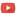KE8QZC | SFW | TSW"How Google converted language translation into a problem of vector space mathematics"
and the paper referenced

Syllabus
Outline of sections

Homework 1 (solution) ($\S$1.1-1.2): pg. 10 #7-14 (solve all systems using matrix method only!), pg. 21-22 #1,2
Homework 2 (solution) ($\S$1.3-1.4): pg. 32-33 #5,6,9,10,11,12,13,27,28; pg.40-41 #1,3,11,12 and the following additional problem:
(A) Solve the matrix equation $A \vec{x}=\vec{b}$ where $A = \left[ \begin{array}{ll} 1 & 0 & 1 \\ 0 & -1 & 1 \\ 0 & 0 & -1 \end{array} \right]$ and $\vec{b}=\left[ \begin{array}{ll} b_1 \\ b_2 \\ b_3 \end{array} \right]$.
Homework 3 (solution)($\S$1.5-1.6): pg.47 #1,3,5,7,9,26,27, pg.54 #7
Homework 4 (solution) ($\S$1.7-1.8): pg.60-61 #1,3,5,7,35,37, pg.68-69 #1,3,7,8,17,19
Homework 5 (solution) ($\S$2.1-2.2): pg.100-101, #1,2,3,7,27, pg.109 #1,2,3,4,8,16,17,18,19
Homework 6 (solution)($\S$2.3-2.4): pg.110 #22,24, pg.114 #5,6,7,8,15,16,17,18, pg.121 #1,2,3,4
Homework 7 (solution) ($\S$3.2,3.3,4.1):pg. 175 #21,22,23, pg.184 #1,5,19,21,23,24 pg.195 #1,3,5,7
Homework 8 (solution) ($\S$4.1,4.2): pg.196 #6,8,9,11,14,20,21,22,33; pg.205-206 #1,3,5,15,30,33,34
Homework 9 (solution) ($\S$4.2,4.3): pg.206-7 #25,26,29,31,32 pg.213 #1,2,3,4,21,22,23,28,33,34
Homework 10 (solution) ($\S$4.4-4.5): pg.222 #1,2,3,4,5,6,7,8,13,14,15,16, pg.229 #1,2,3,4
Homework 11 (solution) ($\S$4.5): pg.229 #5,6,7,9,10,11,13,14,16,17,18,22,24,25,27,29,30
Homework 12 (solution) ($\S$4.6): pg.237 #1,2,3,4,6,8,9,10,11,12,13,14,16,17,18
Homework 13 (solution) ($\S$5.1,5.2): pg. 271 #1,2,3,4,5,9,10,11,12,21,22, pg.279 #1,2,3,4,5,6,7,8

Homework 14 (solution): Homework 14 uses the examples of inner product spaces found in the notes at the bottom of this page. These notes were presented in class, but are also written here since they do not appear in the textbook.
1. Let $H=(\mathbb{R}^3,\langle \cdot,\cdot \rangle)$ be the inner product space of Example 1. Let $\vec{x}=\begin{bmatrix} 3 \\ 2\\ -1\end{bmatrix}$ and $\vec{y}=\begin{bmatrix} 2 \\ 1 \\ 17\end{bmatrix}$. Compute $\langle \vec{x},\vec{y} \rangle$.
2. Let $H=(\mathbb{P}, \langle \cdot,\cdot \rangle)$ be the inner product space of Example 2. Let $\vec{p}(x)=x-1$, $\vec{q}(x)=x^2$. Compute both of the inner products $\langle \vec{p},\vec{q} \rangle$ and $\langle \vec{p},\vec{p} \rangle$ using integration by parts.
3. Use integration by parts to calculate the antiderivative of $f(x)=\log(x)$. (Hint: use $u=\log x$ and $dv=1$. Also recall that $\dfrac{d}{dx} \log x = \dfrac{1}{x}$)
4. Let $H=(C[0,1],\langle \cdot,\cdot \rangle)$ be the inner product space of Example 3. Let $f(x)=\log(x+1)$ and $g(x)=1$. Calculate $\langle f,g \rangle$. Let $h_1(x)=x^2$ and $h_2(x)=\sin(x)$. Calculate $\langle h_1,h_2 \rangle$ (hint: use integration by parts).
5. Let $H=(\ell^1(\mathbb{R}),\langle\cdot,\cdot\rangle)$ be the inner product space of Example 4. Let $\{a_k\}_{k=0}^{\infty} = \left\{ \dfrac{1}{3^k} \right\}_{k=0}^{\infty}$ and $\{b_k\}_{k=0}^{\infty} = \left\{ \dfrac{1}{7^k} \right\}_{k=0}^{\infty}$. Calculate $\left\langle \left\{ a_k \right\}, \left\{b_k\right\} \right\rangle$ (hint: this is a geometric series). Let $\left\{c_k\right\}=\left\{d_k\right\}=\sqrt{\dfrac{1}{k!}}$. Calculate $\langle c_k,d_k \rangle$ (hint:recall the power series $e^x = \displaystyle\sum_{k=0}^{\infty} \dfrac{x^k}{k!}$).
6. Let $H=(\mathbb{C},\langle\cdot,\cdot\rangle)$ be the inner product space of Example 5. Let $\vec{x}=5+4i$ and $\vec{y}=9-11i$. Compute $\langle \vec{x},\vec{y}\rangle$. Let $z_1=21+16i$ and $z_2=\dfrac{11-5i}{2+i}$. Calculate $\langle z_1,z_2\rangle$ (hint: mutltiply $z_2$ by $1=\dfrac{2-i}{2-i}$ to put $z_2$ into the form $z_2=a+bi$; this is similar to "rationalizing denominators").

Homework 15 (solution): Homework 15 uses the definitions from the orthogonality notes on the bottom of this page.
1. Let $(\mathbb{R}^{4 \times 1},\langle \vec{x},\vec{y} \rangle)$ be an inner product space where $\langle \vec{x},\vec{y} \rangle$ denotes dot product.
Show that the vectors $\vec{a}=\begin{bmatrix} 1 \\ 2 \\ 3 \\ 4 \end{bmatrix}$ and $\vec{b}=\begin{bmatrix} -4 \\ -3 \\ 2 \\ 1 \end{bmatrix}$ are orthogonal vectors.
2. Show that the standard basis $\mathscr{B} = \left\{ \begin{bmatrix} 1 \\ 0 \\ 0 \\ 0 \end{bmatrix}, \begin{bmatrix} 0 \\ 1 \\ 0 \\ 0 \end{bmatrix}, \begin{bmatrix} 0 \\ 0\\ 1 \\ 0 \end{bmatrix}, \begin{bmatrix} 0 \\ 0 \\ 0 \\ 1 \end{bmatrix} \right\}$ of $\mathbb{R}^{4 \times 1}$ is a mutually orthogonal set of vectors.
3. Show that the basis $\mathscr{B} = \left\{ \begin{bmatrix} 1 \\ 1 \\ 0 \\ 0 \end{bmatrix}, \begin{bmatrix} 0 \\ 1 \\ 1 \\ 0 \end{bmatrix}, \begin{bmatrix} 0 \\ 0 \\ 1 \\ 0 \end{bmatrix}, \begin{bmatrix} 0 \\ 0 \\ 0 \\ 1 \end{bmatrix} \right\}$ of $\mathbb{R}^{4 \times 1}$ is not a mutually orthognal set of vectors.
4. It was shown in class that $\displaystyle\int_{-\infty}^{\infty} e^{-x^2}dx=\sqrt{\pi}$. Use this fact and integration by parts to compute both $\displaystyle\int_{-\infty}^{\infty} xe^{-x^2} dx$ and $\displaystyle\int_{-\infty}^{\infty} x^2e^{-x^2} dx$. (note: for the first one you can get by with a $u$-substitution and the second one you can do with a clever integration by parts).
5. Consider the vector space $(\mathbb{P},\langle \cdot,\cdot \rangle)$ where the inner product is given by $$\langle p(x),q(x) \rangle = \displaystyle\int_{-\infty}^{\infty} p(x)q(x)e^{-x^2} dx.$$ It can be shown (via methods of problem 4) that the moments in this inner product space are $$\langle 1,1 \rangle=\sqrt{\pi},$$ $$\langle x,1 \rangle=0,$$ $$\langle x^2,1 \rangle=\dfrac{\sqrt{\pi}}{2},$$ $$\langle x^3,1 \rangle = 0,$$ $$\langle x^4,1 \rangle = \dfrac{3\sqrt{\pi}}{4},$$ $$\langle x^5,1 \rangle = 0,$$ $$\langle x^6,1 \rangle = \dfrac{15\sqrt{\pi}}{8}.$$ Use these moments and the "linear in the first argument" property of inner products (noted here) to compute $\langle 4x^2+3x+9,1\rangle$ and $\langle 32x^5-64x^3+24x,1\rangle$.
6. Consider the inner product space $(C(0,L),\langle \cdot,\cdot \rangle)$, where $$\langle f,g \rangle = \displaystyle\int_0^L f(x)g(x) dx.$$ Use the trigonometric identity $$\sin(u)\sin(v) = \dfrac{1}{2} \left[ \cos(u-v) - \cos(u+v) \right]$$ to show that the functions $\left\{ \sin \left( \dfrac{n \pi x}{L} \right) \right\}_{n=1}^{\infty}$ form an orthogonal sequence of functions.
Hint: assume that $m \neq n$ and show that $\left\langle \sin \left(\dfrac{m \pi x}{L} \right), \sin \left( \dfrac{n \pi x }{L} \right) \right\rangle = 0$.

Homework 16: Homework 16 uses the definitions that occur in the notes on projections, Gram-Schmidt, and orthogonal polynomials
1. Consider the inner product space $(C[0,1],\langle \cdot,\cdot \rangle)$ where $$\langle f,g \rangle = \displaystyle\int_0^1 f(x)g(x)x^2 dx.$$ Compute $\mathrm{proj}_{x^2-3x} (5x+2)$ and $\mathrm{proj}_{5x+2}(x^2-3x)$.
2. Consider the inner product space $(\mathbb{R}^{3\times 1},\langle \cdot,\cdot \rangle)$, where $\langle \cdot,\cdot \rangle$ denotes the dot-product. Consider the finite sequence of vectors $(\vec{v}_n)_{n=1}^{3}$ given by the formula $\vec{v}_n = \begin{bmatrix} n \\ n^2 \\ n^3 \end{bmatrix}$, so we have $v_1=\begin{bmatrix} 1 \\ 1 \\ 1 \end{bmatrix}, \vec{v}_2 = \begin{bmatrix} 2 \\ 4 \\ 8 \end{bmatrix}, \vec{v}_3 = \begin{bmatrix} 3 \\ 9 \\ 27 \end{bmatrix}$. It is clear that this sequence is not an orthogonal sequence of vectors. Apply the Gram-Schmidt process to orthogonalize this sequence.
3. Find the orthonormal sequence associated with the answer to Problem 2.
4. Consider the inner product space $(\mathbb{P},\langle \cdot,\cdot \rangle)$ where $\langle \vec{p},\vec{q} \rangle = \displaystyle\int_{-1}^1 \vec{p}(x)\vec{q}(x) dx$. Find the moments $\langle 1,1\rangle, \langle x,1 \rangle,$ and $\langle x^2, 1 \rangle$.
5. Consider the inner product space from Problem 4. Consider the sequence of vectors $(x^n)_{n=0}^{\infty} = (1,x,x^2,\ldots)$. Why is this sequence not a sequence of orthogonal vectors?
6. Consider the inner product space and sequence from Problem 5. Apply the Gram-Schmidt process to the sequence $(x^n)_{n=0}^{\infty}$ to find the first four polynomials polynomials orthogonal with respect to $\langle \cdot,\cdot \rangle$. (note: these polynomials are called Legendre polynomials)
7. Normalize the the four polynomials found in Problem 6 so that they would fit into a sequence of orthonormal polynomials.

Other stuff
1. Using linear systems to balance a chemical equation
2. Notes on inner product spaces
3. Notes on orthogonality
4. Projections, Gram-Schmidt, orthogonal polynomials